﻿ Formula Stock Illustrations – 70,672 Formula Stock Illustrations, Vectors & Clipart - Dreamstime
Filter search results
Sort by
Content type
Image orientation
Color composition
People
Number of people
Gender
Age group
Ethnicity
More filters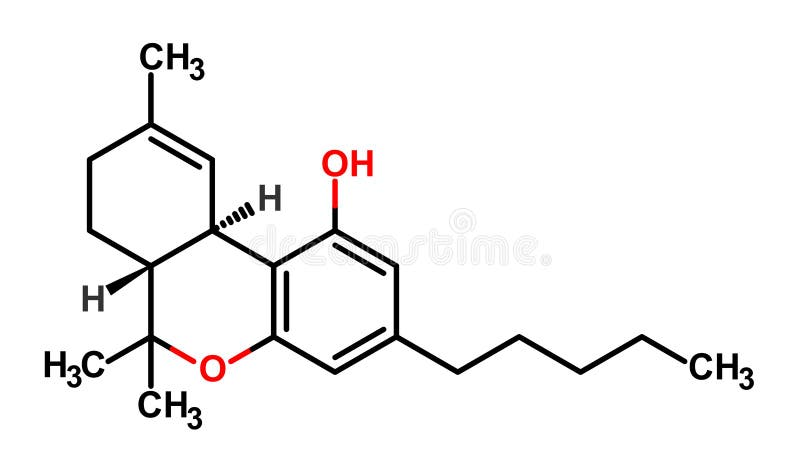THC formula. Structural formula of Tetrahydrocannabinol (THC), the psychoactive constituent of the cannabis plantCreation New Formula Drugs from Plant Materials. Strategy and Tehnology Creating New Drug from Ecological Herbs and Plants. Scientists Put in Test Tubes and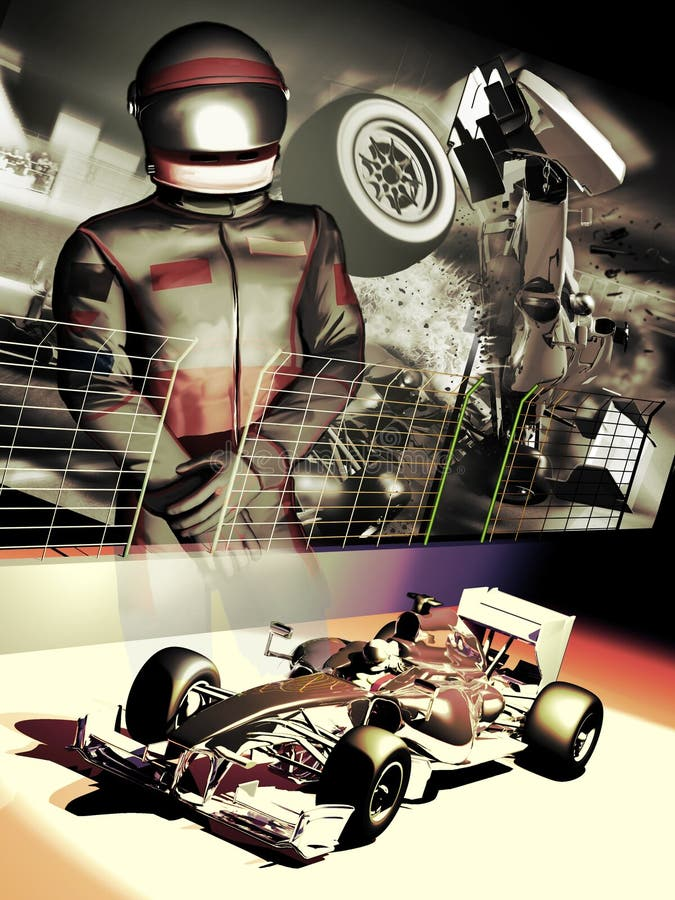Formula one driver. Poster presentation of a formula one driver, his car, and an dreadful accident in the background, as a reminder of a crucial moment in his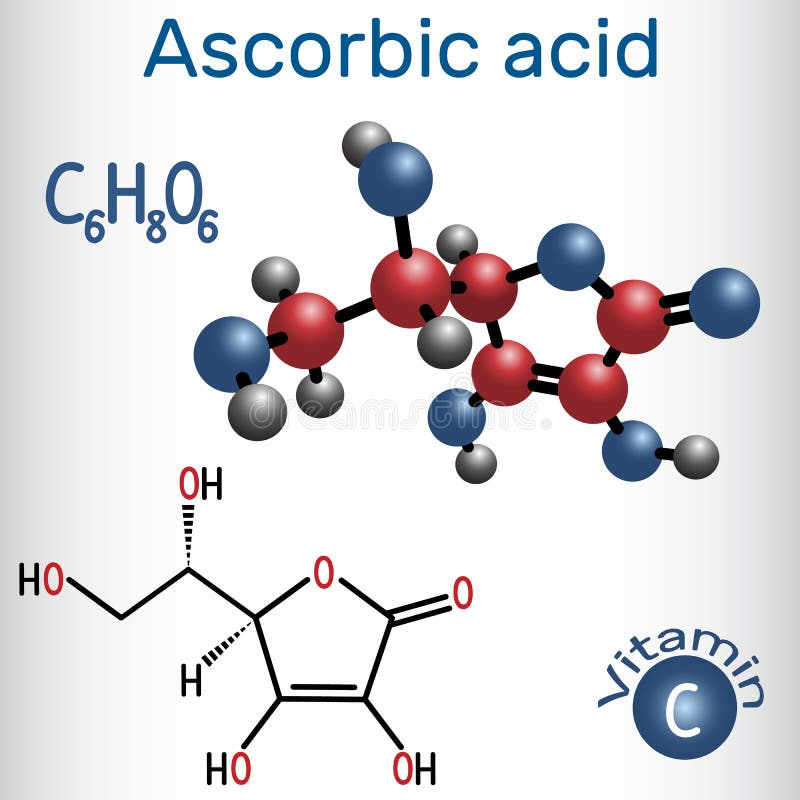Ascorbic acid vitamin C. Structural chemical formula and molec. Ule model. Vector illustration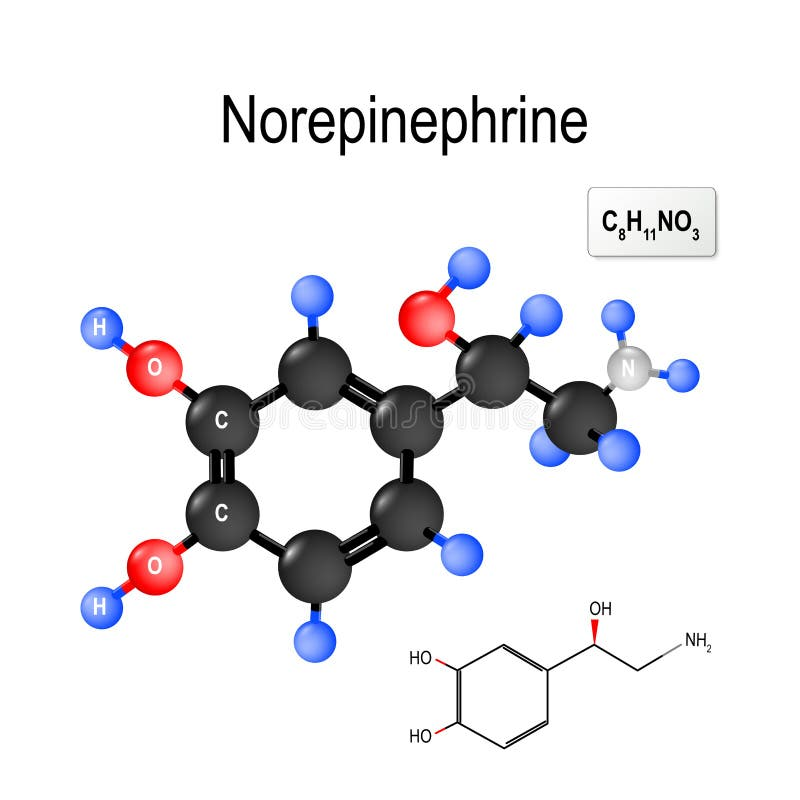Norepinephrine. Structural chemical formula and model of molecule of noradrenalin. Norepinephrine noradrenaline is an organic chemical that functions in the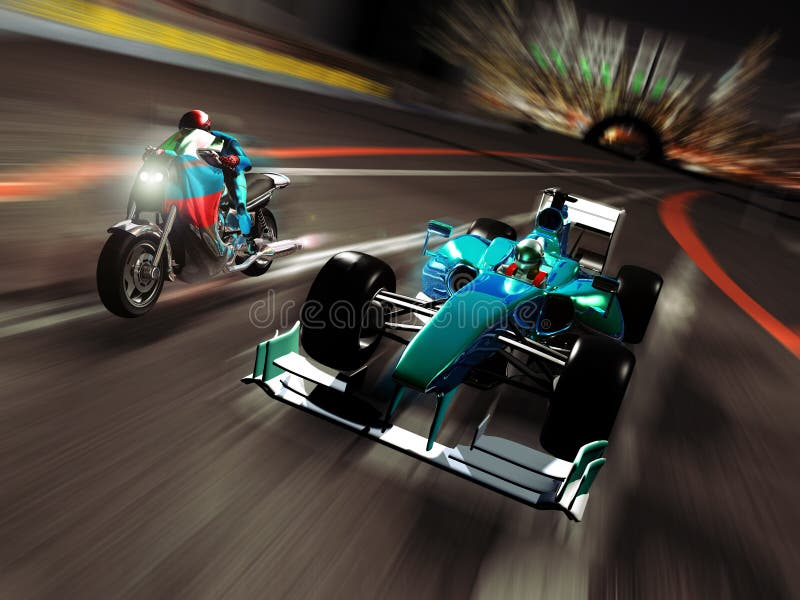Motorcycle versus formula one. On a race circuit, competition between a powerful motorcycle and a formula one car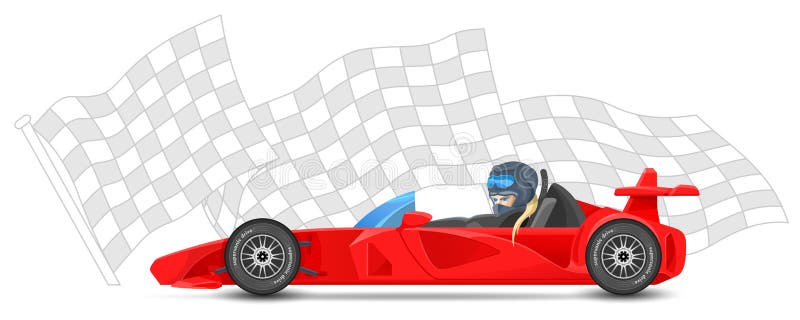Red race car side view, formula 1, on sports finish flag background. Bolides sport. Red race car side view, formula 1 one, on sports finish flag background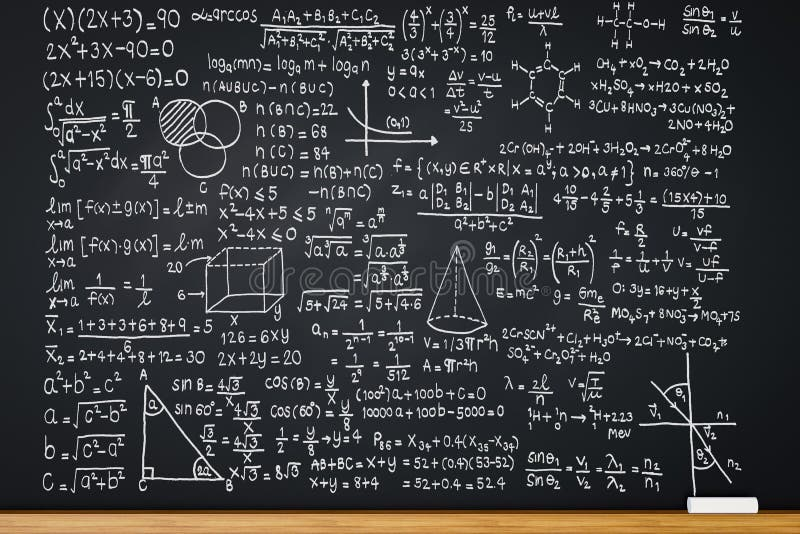Blackboard with math formula. 3d rendering blackboard with math formula or education background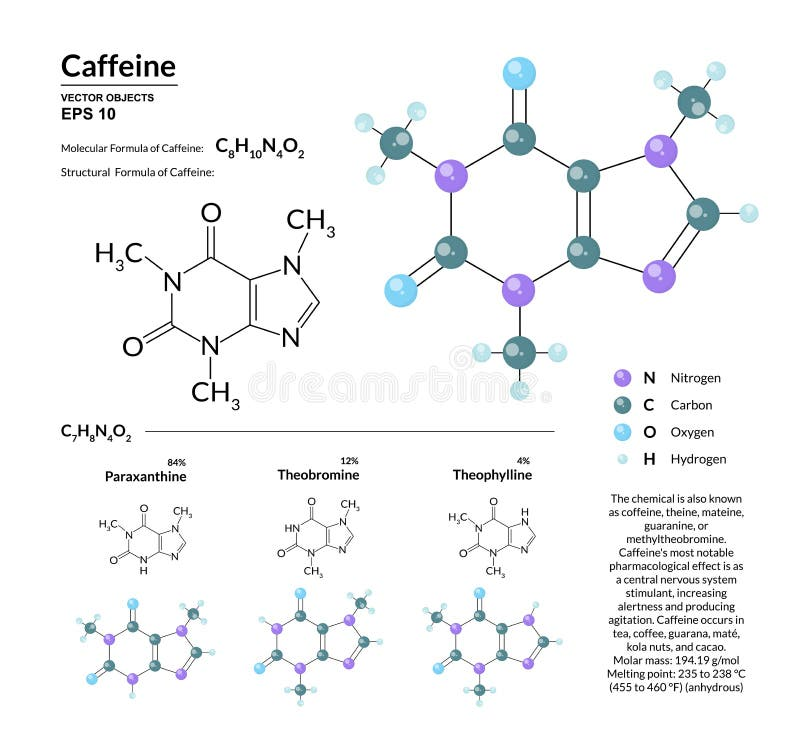Structural chemical molecular formula and model of caffeine. Atoms are represented as spheres with color coding. Isolated on background. 2d or 3d visualization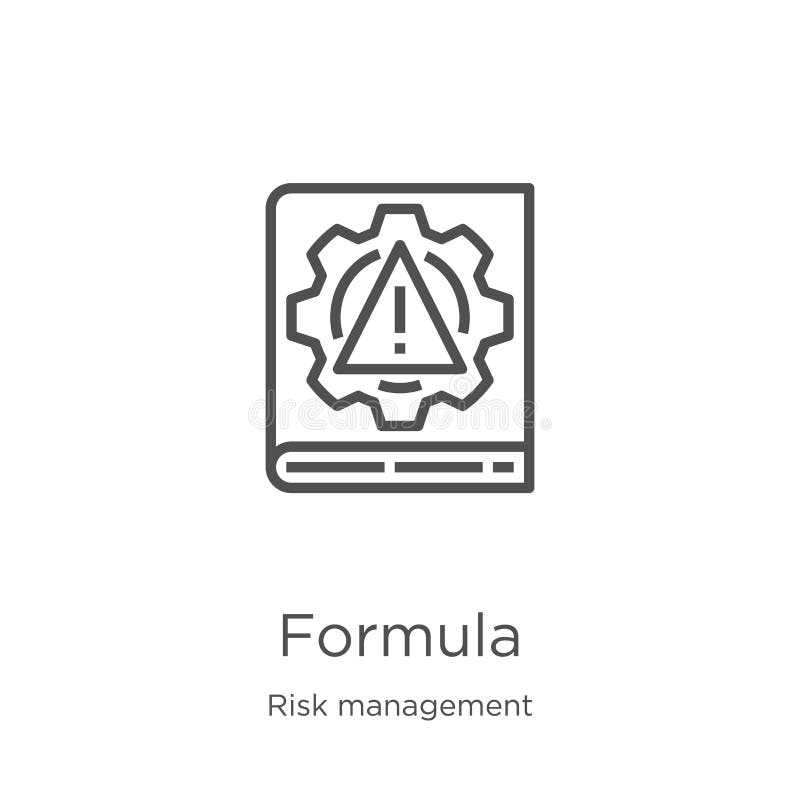Formula icon vector from risk management collection. Thin line formula outline icon vector illustration. Outline, thin line. Formula icon. Element of riskFormula 1 icon in trendy design style. formula 1 icon isolated on white background. formula 1 vector icon simple and modern flat. Symbol for web site, mobileCannabidiol CBD - structural chemical formula and molecule. Model. Active cannabinoid in cannabis, has antipsychotic effects. Sheet of paper in a cage. VectorFormula 1 Racing Car. Isolated on white backgroundSplash Water Formula H2O. H2O Splash Water Formula isolated on a white backgroundFormula One. Driver And Racing Car Illustration, VectorFormula for Healthy Heart. Vector Infographic Illustration of Formula for a Healthy HeartFormula racing car. DesignWireframe formula 1 car. A wireframe formula 1 car on a white backgroundDopamine. Structural chemical formula and model of molecule. Dopamine DA, dihydroxyphenethylamine is an organic chemical of the catecholamine and phenethylamineFormula 1 Racing Car. Isolated on white backgroundChemical formula of Cortisol. The chemical formula of Cortisol3D pin with formula one. Insignia isolated on whiteStructural chemical formula and model of molecule of adrenalin. Adrenaline epinephrine is a hormone, neurotransmitter, and medication. Produced by the adrenalStructural chemical formula and model of molecule of Serotonin. Serotonin is a neurotransmitter contributor to feelings of well-being and happiness. StructuralGlycerol glycerine molecule. Structural chemical formula and m. Olecule model. Vector illustrationFormula e=mc2/. Albert Einstein portrait with chalk on chalkboard and his famous formulaResveratrol molecule. It is natural phenol, phytoalexin, antioxidant. Structural chemical formula and molecule model. Vector illustrationO3 Ozone formula. Illustration of the Ozone molecular formula on white background3D render - formula one chassis structural analysis. 3D render illustration of a formula one chassis structural analysis. The composition is isolated on a whiteFormula One. Colored illustration, vectorThe percentage of different types of white blood cells. Leukocyte formula. Neutrophils, monocytes, lymphocytes. Eosinophils, basophils. Cell killers. ImmunityThe chemical formula background seamless. The vector image The chemical formula background seamlessFormula 1 concept SET 1. 5 views of conceptual formula 1 car, easy to colorizeFormula 1 Car. Racing speeding in the trackCoenzyme Q10 serum essence drops formula. Coenzyme Q10 serum essence gold drops with dropper. Skin care collagen hyaluronic moisture formula treatment designChemical formula of water made of water splashes on black. BackgroundWave physics science theory law and mathematical formula equation, doodle handwriting and frequencies model icon in white isolate. D background paper used forNitric Acid formula. Illustration of Nitric Acid formula on dark backgroundAbstract blue formula racing car. Isolated vector silhouette. Race carFormula 1 icon set, sport icons and sticker - 3. Formula 1 on a high quality icon setFormula 1 icon set, sport icons and sticker - 2. Formula 1 on a high quality icon setHand writing maths formula on seamless blackboard. Hand writing mathematics formula on seamless blackboard as backgroundH2O. Formula of water. H2O. Formula of water on white isolated background. 3dFormula one car. 3D render of a formula one car on a motion backgroundEnergy Formula. Albert Einsteins energy formula written on blackboardFormula of water. H2O. Formula of water on white backgroundBicarbonate anion HCO3 - structural chemical formula and mol. Ecule model. Vector illustrationH2O. Formula of water. H2O. Formula of water on white isolated background. 3dThe acetaminophen formula. Illustration of the structural formula of acetaminophenSulfuric Acid formula. Illustration on the dark backgroundSulfuric acid formula. Freehand illustration of the Sulfuric acid formula on dark backgroundChemical formula on chalkboard. Chemical formula on black chalkboardHand writing maths formula on seamless greenboard. Hand writing mathematics formula on seamless greenboard as backgroundFormula one car. 3D render of a formula one car on a motion backgroundHand writing maths formula on chalkboard. Hand writing maths formula on green boardStructural formula of taurine. Isolated on white backgroundSucrose molecule with chemical formula. Isolated on white backgroundValine skeletal formula. The Valine amino acid molecule. Skeletal formulaPhosphoric acid formula. Freehand illustration of the Phosphoric acid formula on dark backgroundCholesterol molecule with chemical formula. Isolated on white backgroundThe molecular formula of oxygen. Illustration of the molecular formula of oxygen on white backgroundLeucine skeletal formula. The Leucine amino acid molecule. Skeletal formulaCH4 Methane formula icon. On dark background with labelStructural formula of testosterone. (male sex hormone) isolated on white backgroundPhysical formula on blackboard. Albert Einsteins physical formula on blackboardH2O Chemical formula icon. On colorful background with labelFormula 1. Vector illustration of formula 1. racing carResveratrol molecule. It is natural phenol, phytoalexin, antioxidant. Structural chemical formula and molecule model. Sheet of pa. Per in a cage. VectorVintage sport racing car flat formula vector vehicle auto illustration. IconVitamin d formula is ensuring the absorption of calcium and phosphorus from food. Vitamin D D3, cholecalciferol molecule. Skeletal formula. vitamin d formula is3d render of molecular model and formula of vitamin C. 3d render of molecular model with molecular formula of vitamin C over white backgroundButton with energy formula on abstract background. With clipping pathCapsaicin molecule with chemical formula. Capsaicin (C18H27NO3) molecule with chemical formula isolated on white backgroundButane formula illustration. Freehand style illustration of the butane formulaButane formula illustration. The illustration of the butane structural formulaOctane formula illustration. Freehand style illustration of the octane formulaOctane formula illustration. The illustration of the octane structural formulaMethanol structural formula. The Methanol structural formula on dark backgroundHexane formula illustration. The illustration of the hexane structural formulaHexane formula illustration. Freehand style illustration of the hexane formula